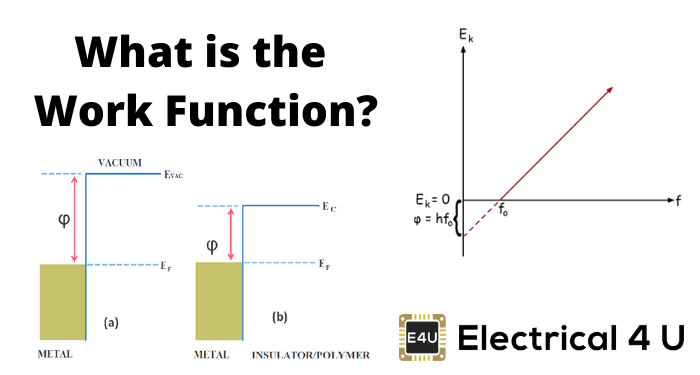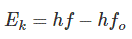# Work Function: Equation & Relation To Threshold Frequency

Contents## Work Function Definition

The work function (or workfunction) is defined as the minimum amount of thermodynamic work (i.e. energy) required to remove an electron from a solid to a point in the vacuum immediately outside the solid surface. The symbol for work function is Φ (uppercase Phi of the Greek alphabet).

## Work Function in Classical Physics

The concept of a work function can be explained in both classical physics or quantum mechanics. As per classical physics, when an electron tries to escape from a metal surface, it leaves a positive image behind in the metal surface.

Due to the attraction of this positive image, the negative electron turns back to metal surface hence cannot leave the metal crystal permanently. But to overcome this attraction force an electron requires sufficient energy supplied from outside usually from external light sources. The minimum energy required just to escape an electron from a metal surface is known as work function.

## Work Function in Quantum Physics

Work function can also be explained and defined by quantum physics. For that, we first have to know some basic features.

1. Sir Albert Einstein said that light is in the form of a beam of a huge number of discrete energy packets called photons. The energy contained in each photon is hf. Where h is Planck Constant and f is the frequency of light.
2. Now when the light strikes on a metal surface electrons on the surface of the metal get energy from the light and get emitted from the surface. This phenomenon is classically termed as photoemission.
3. As the energy of one photon Ephoton = hf, the energy of each photon depends upon the frequency of light. Hence, the frequency is only variable upon which energy of photon rather light depends., It is found that there is no photoemission from a metal surface below a certain frequency of light. Hence, for photoemission minimum frequency of incident light is required.
4. If the frequency of light is higher than that above-mentioned minimum frequency, the extra energy of photon will be converted to kinetic energy of the emitted electron. Hence, how fast the electron will be emitted from the surface of the metal depends upon the frequency of incident light. Not on the intensity (Brightness of the light).
5. But when an intensity of incident light gets increased without changing its frequency. Obviously, the number of photons strike on the metal surface increases hence more emitted electrons will be produced, but the kinetic energy of each electron will be unchanged as the frequency of incident light is fixed, in that case.
6. ### Threshold Frequency

Hence after a certain minimum frequency of incident light, the electrons start emitted from a metal surface. Above this frequency, the kinetic energy of the emitted electron is directly proportional to incident light frequency. But below this minimum frequency, there will be no kinetic energy in the electrons. This is called threshold frequency.

7. The frequency of incident light below which no photoemission on a metal surface gets initiated is called threshold frequency for that metal. The value of threshold frequency is different for different metals.

## Graphical Representation of Work Function

Now, If we graphically represent the above points we will get the graph below,Here, the vertical axis represents the energy of an electron, and the horizontal axis represents the frequency.

After frequency fo Hz, the kinetic energy of electrons start increasing proportionally with frequency.

Below, frequency fo or below energy hfo [ h is Planck Constant ] there will be no kinetic energy i.e. no emission of an electron. This amount of energy i.e. hfo is known and defined as work function φ.

## Work Function Formula

The gain of kinetic energy of an electron is the difference between incident photon energy and work function of the metal or material and that can be represented asWhere, φ is the work function of the metal and Ek is the kinetic energy gain of the electron. The equaltion can be rewritten asWhere fo is threshold frequency of the metal.

## Work Function of Metals

Let us show a table for work function of various commonly used metals

 Metals Work Function in eV Al (Aluminum) 4.3 Ti (Titanium) 4.33 V (Vanadium) 4.3 Cr (Chromium) 4.5 Mn (Manganese) 4.1 Fe (Iron) 4.7 Co (Cobalt) 5 Ni (Nickel) 5.15 Nb (Niobium) 4.3 Mo (Molybdenum) 4.6 Ru (Ruthenium) 4.7 Rh (Rhodium) 4.98 Hf (Hafnium) 3.9 Ta (Tantalum) 4.25 W (Tungsten) 4.55 Re (Rhenium) 4.96 Os (Osmium) 4.83 Ir (Iridium) 5.27 Au (Gold) 5.1
Want To Learn Faster? 🎓
Get electrical articles delivered to your inbox every week.
No credit card required—it’s 100% free.